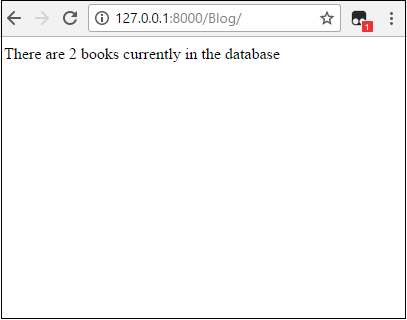﻿ How to Count All Objects of a Database Table in Django ﻿# How to Count All Objects of a Database Table in DjangoIn this article, we show how to count all objects of a database table (a model) in Django.

This allows us to know how many rows of data have been inserted into a database table.

Let's say we have a database table called Book.

And this database table stores books (book objects).

We can obtain how many books there are in the table using built-in Python functions.

So if you have a database table named Book and you want to find out how many books there in it, the following line of code, shown below, could do so.

Now in the above code, the variable count contains how many book objects there are in the Book database table.

So let's through the entire code you would have in the views.py file, to get a broader view of how this works.

### views.py File

So now let's go through the entire code you would have in the views.py file.

So we said that we created a Book database table in the models.py file in the current app that we're working with.

So, in our views.py file, we have to import the database table from the models.py file in order to access it.

We then create our count variable which holds how many objects there are in our Book database table.

We then create a context dictionary and pass into the template file the count variable.

So, quickly going over the code, we import render, which is able to render HTML template files or other template files.

We have import our database table, Book, from models.py in order to access it.

We then create our function, in this case named showthis().

We always must pass in request as a parameter.

We then create a variable named count and set it equal to, Book.objects.all().count()

This gets the count of all of the book objects in our Book database table.

We then create a context dictionary, which in which we pass in the variable, count.

We then render the context dictionary into the home.html page.

This passes the count variable into the home.html page.

### home.html

Lastly, we just have to create the home.html page template file.

So this will be a very basic file in which we just want to print out how many books are in the database table.

So we can do this through the following code below.

So we just have a basic HTML file.

In the body section of the HTML file, where most of the content that a user sees goes, we have a paragraph tag that tells us how many books are currently in the database table.

Since count is a variable, we render in Django using double opening and closing curly braces. This renders a variable in Django.

I put in 2 objects in the Book database table that can be can be seen here: Book Database Table in Django

Running the code gives me the following output.And you can see, due to the fact that we have 2 objects in the Book database table, the output tells us this.

So this is how we can count all of the objects in a database table in Django.

Related Resources

How to Randomly Select From or Shuffle a List in Python